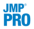Publication date: 11/10/2021

## BoostingBoosting is the process of building a large additive neural network model by fitting a sequence of smaller models. Each of the smaller models is fit on the scaled residuals of the previous model. The models are combined to form the larger final model. The process uses validation to assess how many component models to fit, not exceeding the specified number of models.

Boosting is often faster than fitting a single large model. However, the base model should be a 1 to 2 node single-layer model. The benefit of faster fitting can be lost if a large number of models is specified.

Use the Boosting panel in the Model Launch control panel to specify the number of component models and the learning rate. Use the Hidden Layer Structure panel in the Model Launch control panel to specify the structure of the base model.

The learning rate must be 0 < r 1. Learning rates close to 1 result in faster convergence on a final model, but also have a higher tendency to overfit data. Use learning rates close to 1 when a small Number of Models is specified.

As an example of how boosting works, suppose you specify a base model consisting of one layer and two nodes, with the number of models equal to eight. The first step is to fit a one-layer, two-node model. The predicted values from that model are scaled by the learning rate, then subtracted from the actual values to form a scaled residual. The next step is to fit a different one-layer, two-node model, where the response values are the scaled residuals of the previous model. This process continues until eight models are fit, or until the addition of another model fails to improve the validation statistic. The component models are combined to form the final, large model. In this example, if six models are fit before stopping, the final model consists of one layer and 2 x 6 = 12 nodes.

Caution: You cannot use boosting with a two-layer neural network. If you specify any non-zero values in the second layer and also specify boosting, the second layer is ignored.